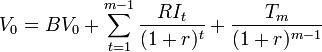# Residual Income Valuation (RIV)

Appraisal Print Email

## Definition of Residual Income Valuation

Residual income valuation (RIV) which is also known as residual income method or residual income model (RIM) is an approach to or method of equity valuation which properly accounts for the cost of equity capital. The word ‘residual’ refers to any opportunity costs in excess which is measured as compared to the book value of the shareholders’ equity and the income that a firm generates after accounting for the true cost of capital is then the residual income. This approach is largely similar to the MVA/EVA based approach having similar advantages and logic.

## Concept of Residual Income Valuation

The basic idea behind this approach is that a rate of return is required by investors from their resources which are under the management of the firm, provide compensation for their opportunity cost and account for the level of risk. This rate of return is considered the cost of equity and a formal equity cost has to be subtracted from net income. Again, for creating shareholder value, management should be capable of generating returns which is at least equal to this cost. Therefore, even if the income statement of a company report a profit, it can be actually unprofitable economically. Thus, it is possible that a value may be negative in this case, even though it is positive when traditional discounted cash flow approach is applied.

## Calculating Residual Income

Usually, for calculating cost of capital, CAPM is used. However, there are other approaches like APT which are also used. The formula for calculating residual income is as follow:

Equity Charge = Equity Capital x Cost of Equity

Residual Income = Net Income – Equity Charge

Formula for valuation

In residual income approach, a company’s stock value can be calculated as sum total of its book value and its expected future residual income’s present value which is discounted at cost of equity, r. the general formula is:This formula is used when it is assumed that the company will achieve maturity or constant growth. Here, for calculating the corresponding terminal value, mainly perpetual growth model is used. The terminal value when it is assumed long run constant growth g from year m is:Therefore, the residual income valuation is:Residual income valuation is a powerful tool for estimating the intrinsic value of a company's stock based on its ability to create economic profit. It provides a more comprehensive and accurate measure of a company's value than traditional accounting methods, and can help investors make informed decisions about whether to invest in a particular company.